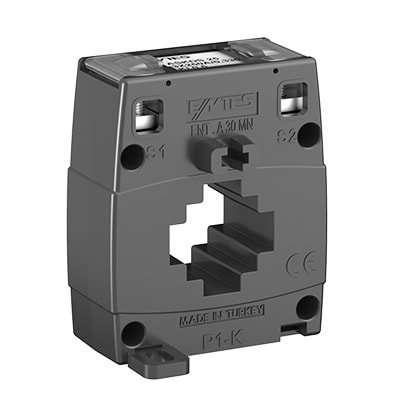# ENT.A30MN Series

 Secondary Current 5A 1A Primary Current (A) Nominal Power(VA) Class Class 3 1 0,5 3 1 0,5 40 1 ✓ X X X X X 50 1 X ✓ X X X X 60 1 X X X ✓ X 1,5 X ✓ X X X X 75 1,25 X X X ✓ X 1,5 X ✓ X X X X 80 1,5 X X X X ✓ X 2,5 X ✓ X X X X 100 1,5 X X ✓ X X X 2,5 X ✓ v X ✓ X 125 1,5 X X ✓ X X X 2,5 X ✓ X X ✓ X 150 2,5 X ✓ X X X ✓ 2,5 X X ✓ X X 200 2,5 X X ✓ X X ✓ 250 2,5 X X ✓ X X 5 X X X X ✓ 300 5 X X ✓ X X ✓ 400 5 X X ✓ X X X 500 5 X X ✓ X X X 600 5 X X ✓ X X ✓
OTHER PRODUCTS
SUPPORT AND DOCUMENTS
Get Offer
Let Us Call You